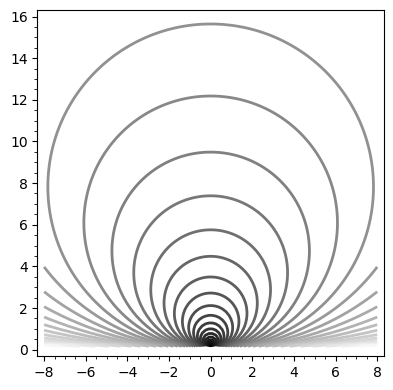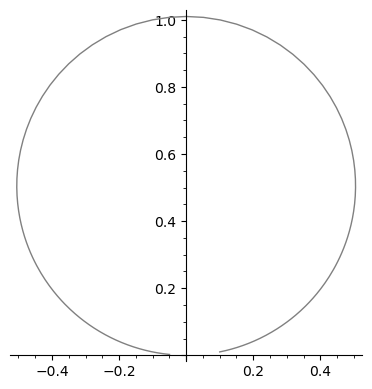# 18. Integral curves¶

This notebook is part of the Introduction to manifolds in SageMath by Andrzej Chrzeszczyk (Jan Kochanowski University of Kielce, Poland).

In :
version()

Out:
'SageMath version 9.6, Release Date: 2022-05-15'

Let $X$ be a vector field on a smooth manifold $M$. A curve $\gamma : I → M$ is an integral curve of $\mathbf{X}$ if

\begin{equation} \displaystyle \gamma'_t = X_{\gamma(t)} , \mbox{ for } t ∈ I. \tag{18.1} \end{equation}

Let us recall that $\gamma'_t\$ is the tangent vector to $\gamma$ at $t$ (defined in (8.9)).

If $\gamma(0) = x\in M$, we say that the curve $\gamma$ starts at $x$.

Let ϕ be a one-parameter group of transformations on $M$ (defined in a previous notebook) and let $X$ be its infinitesimal generator. If we put $y = ϕ_{t_0} (x) = ϕ(x, t_0 )$, we get

$$ϕ_y (t) = ϕ(y, t) = ϕ(ϕ(x, t_0 ), t) = ϕ(x, t_0 + t) = ϕ_x (t_0 + t).$$

The tangent vector to the curve $\phi_y$ at $t=0$ satisfies $$(ϕ_y )'_0 ( f ) =\frac{d}{dt}(f (ϕ_y (t)))\Big|_{t=0}= \frac{d}{dt}(f (ϕ_x (t+t_0)))\Big|_{t=0}= \frac{d}{ds}(f (ϕ_x (s)))\Big|_{s=t_0}=(ϕ_x)'_{t_0} ( f ),$$
for any $f ∈ C^∞ (M)$, and therefore $(ϕ_x )'_{t_0} = (ϕ_y )'_0 = X_y = X_{ ϕ_x (t_0 )}$.

We have checked that:
if $y = ϕ_x (t_0 )$, for some $t_0 ∈ R$ then $(ϕ_x )'_{t_0} = (ϕ_y )'_0$ and, therefore, $(ϕ_x )'_{t_0} = X_{ϕ_x (t_0 )}$ ; that is, the tangent vector to the curve $ϕ_x$, at any point of the curve, coincides with the value of the infinitesimal generator of ϕ at that point.

Thus if $ϕ$ is a one-parameter group of transformations and $X$ is its infinitesimal generator, then the curve $ϕ_x$ is an integral curve of X that starts at $x$.

Let us recall that if $\phi=(x^1,\ldots,x^n)$ are local coordinates on a smooth manifold $M$, $\gamma$ is a smooth curve in $M$, then from (8.10) it follows $$\gamma'_t=\frac{d}{dt}(x^i\circ\gamma)\Big|_t\frac{\partial}{\partial x^i}\Big|_{\gamma(t)}.$$

Since from $\ \ (Xf)(p)=X_p(f)\$ we obtain $X(x^i)(\gamma(t))=X_{\gamma(t)}(x^i)$, then by (8.5) the right hand side of (18.1) can be written as $$X_{\gamma(t)} = X_{\gamma(t)}(x^i)\frac{∂}{∂ x^i}\Big|_{\gamma(t)}= X(x^i)(\gamma(t)) \frac{∂}{∂ x^i}\Big|_{\gamma(t)}= (X^i\circ\gamma)(t) \frac{∂}{∂ x^i}\Big|_{\gamma(t)},$$ where $X^i=X(x^i).$
The last two equations imply, that the integral curves $\gamma$ of $X$ defined by (18.1) satisfy the system of ordinary differential equations \begin{equation} \frac{d}{dt}(x^i\circ\gamma)=X^i\circ\gamma,\quad i=1,\ldots,n. \tag{18.2} \end{equation}

The right-hand side of (18.2) ca be written in the form $$(X^i ◦ \gamma)(t) = (X^i ◦ φ^{−1} ) φ(\gamma(t))\\ = (X^i ◦ φ^{−1} ) (x^1 (\gamma(t)), . . . , x^n (\gamma(t)))\\ = (X^i ◦ φ^{−1} ) ((x^1 ◦ \gamma)(t),..., (x^n ◦ \gamma)(t)),$$ so (18.2) is equivalent to \begin{equation} \frac{d(x^i\circ\gamma)}{dt}= (X^i ◦ φ^{−1} )(x^1 ◦ \gamma, . . . , x^n ◦ \gamma). \tag{18.3} \end{equation} If we put $f^i = X^i ◦ φ^{−1}$ and replace $x^i ◦ \gamma$ by $x^i$ we obtain the simplified form of the system (18.3) \begin{equation} \frac{dx^i}{dt}=f^i(x^1,\ldots,x^n),\quad i=1,\ldots,n. \tag{18.4} \end{equation}

For a smooth vector field $X$, on a smooth manifold $M$, given $x\in M$ there exist a unique integral curve $\gamma$ of the vector field $X$ starting at $x$, defined in some interval $I\subset R$. Define

\begin{equation} \phi(x,t)=\gamma(t). \tag{18.5} \end{equation}

We want to check that if the integral curves of $X$ are defined for all $t\in R$, then $\phi$ is a one-parameter group of transformations.

Consider the curve $\chi$ defined by $$\chi(t)=\gamma(t+s),\quad s-\mbox{fixed}.$$ The curve $\chi$ is an integral curve of $X$, since for $f\in C^\infty(M)$

$$\chi'_t(f)=\frac{d(f\circ\chi)}{dt}\Big|_t =\lim_{h\to 0}\frac{f(\chi(t+h))-f(\chi(t))}{h}\\ =\lim_{h\to 0}\frac{f(\gamma(t+h+s))-f(\gamma(t+s))}{h} =\gamma'_{t+s}(f)=X_{\gamma(t+s)}(f)=X_{\chi(t)}(f).$$

The curve $\chi$ starts at $\chi(0)=\gamma(s)$ and the curve $\phi(\gamma(s),t)$ also starts at $\gamma(s)$. By the uniqueness of the integral curves of smooth vector fields we have

$$\chi(t)=\phi(\gamma (s),t)=\phi(\phi(x,s),t).$$

We have also $$\chi(t)=\gamma(t+s)=\phi(x,t+s),$$ so \begin{equation} \phi(\phi(x,s),t)=\phi(x,t+s). \tag{18.6} \end{equation}

Thus $\phi$ defines a one-parameter group of transformations.

Example 18.1

For $\ X=y\frac{\partial}{\partial x}+x\frac{\partial}{\partial y}$, the system (18.4) takes the form $$\frac{dx}{dt}=y,\quad \frac{dy}{dt}=x.$$

Let us solve the system with Sympy.

In :
from sympy import *                   # import Sympy
init_printing()                       # use latex to output results
t,x0,y0 = symbols('t,x0,y0')          # Sympy variables
x = Function('x')                     # Sympy function x(t)
y = Function('y')                     # Sympy function y(t)
ics = {x(0): x0,y(0):y0}              # initial conditions
# solve ODE system in Sympy:
sol = dsolve([x(t).diff(t)-y(t), y(t).diff(t) -x(t)],ics=ics)
import sympy                          # "sympy" not defined previously
(sol.rhs).rewrite(sympy.sin).simplify()  # use Sympy rewrite method
# to simplify first component

Out:
$\displaystyle x_{0} \cosh{\left(t \right)} + y_{0} \sinh{\left(t \right)}$

(first component of the solution),

In :
(sol.rhs).rewrite(sympy.sin).simplify()  # do the same for second comp.

Out:
$\displaystyle x_{0} \sinh{\left(t \right)} + y_{0} \cosh{\left(t \right)}$

(second component).

Example 18.2

Check, that the integral curves from previous example define one-parameter group of transformations.

Let us define the components of the one-parameter group of transformations $\phi$ corresponding to the vector field

$$X=(𝑥_0\cosh(𝑡)+𝑦_0\sinh(𝑡))\frac{\partial}{\partial x} +(𝑥_0\sinh(𝑡)+𝑦_0\cosh(𝑡))\frac{\partial}{\partial y}$$

from the previous example, using (18.5):

In :
reset()
var('t,s,x,y')                    # symbolic variables
# \phi[Tab] gives the greek letter phi
ϕ1(x,y,t)=x*cosh(t) + y*sinh(t)   # define ϕ1
ϕ2(x,y,t)=x*sinh(t) + y*cosh(t)   # define ϕ2


Now let us compute the difference between the right and left hand sides of the first components of (18.6):

In :
a1=ϕ1(x,y,t+s).trig_expand().expand()  # right hand side of (18.6) for ϕ1
b1=ϕ1(ϕ1(x,y,t),ϕ2(x,y,t),s).expand()  # left hand side of (18.6) for ϕ1
a1-b1                                  # rhs-lhs

Out:
0

The same for the second components:

In :
a2=ϕ2(x,y,t+s).trig_expand().expand()  # right hand side of (18.6) for ϕ2
b2=ϕ2(ϕ1(x,y,t),ϕ2(x,y,t),s).expand()  # left hand side of (18.6) for ϕ2
a2-b2                                  # rhs-lhs

Out:
0

We have checked that (18.6) is fulfilled.

Example 18.3

For the vector field $\displaystyle X = x^2 \frac{∂}{∂ x} + 2x y \frac{∂}{∂ y}$ the system (18.4) takes the form $$\frac{dx}{dt}=x^2,\quad \frac{dy}{dt}=2xy.$$
Solve it.

In :
from sympy import *              # import Sympy
x0=symbols('x0')                 # initial value for x in Sympy
y0=symbols('y0')                 # initial value for y in Sympy
t = symbols('t')                 # symbolic variable t in Sympy
x = Function('x')                # function x(t) in Sympy
y = Function('y')                # function y(t) in Sympy
ics = {x(0): x0,y(0):y0}         # initial conditions
# solve ODE system in Sympy:
sol = dsolve([x(t).diff(t) - x(t)**2,
y(t).diff(t) - 2*x(t)*y(t)],ics=ics)


The system has the following solution:

In :
init_printing()
list(map(simplify,sol))

Out:
$\displaystyle \left[ x{\left(t \right)} = - \frac{x_{0}}{t x_{0} - 1}, \ y{\left(t \right)} = \frac{y_{0}}{\left(t x_{0} - 1\right)^{2}}\right]$

The expression is not defined for $t = 1/x_0$ , and therefore we are not dealing with a one-parameter group of transformations (by definition defined for $t\in R$), despite the fact that $X$ is smooth.

Example 18.4

Show that the integral curve from the previous example defines a local version of one parameter group of transformations $\phi$ with $t$ in a sufficiently small neighborhood of zero.

Define components of $\phi$:

In :
reset()
var('t,s,x,y')                  # symbolic variables
ϕ1(x,y,t)=-x/(t*x - 1)          # define ϕ1
ϕ2(x,y,t)=y/(t*x - 1)**2        # define ϕ2


next, the right and left hand sides of (18.6):

In :
a1=ϕ1(x,y,t+s).normalize()     # right hand side of (18.6) for ϕ1
b1=ϕ1(ϕ1(x,y,t),ϕ2(x,y,t),s).normalize()  # lhs of (18.6) for ϕ1

a2=ϕ2(x,y,t+s).normalize()     # right hand side of (18.6) for ϕ2
b2=ϕ2(ϕ1(x,y,t),ϕ2(x,y,t),s).normalize()  # lhs of (18.6) for ϕ2


and the differences between the right and left hand sides

In :
a1-b1,a2-b2                    # lhs - rhs

Out:
(0, 0)

So (18.6) is fulfilled for sufficiently small $t$ and $s$.

Example 18.5

For $\displaystyle X = \frac{1}{2} (x^2 − y^2 ) \frac{∂}{∂ x} + x y \frac{∂}{∂ y}$ on $M = \{(x, y) ∈ R^2 : y > 0\}$ we have $$\frac{dx}{dt}=\frac{1}{2}(x^2-y^2),\quad \frac{dy}{dt}=xy.$$ Solve the system.

The system implies the single differential equation $$\frac{dy}{dx} = 2x y/(x^2 − y^2 ).$$ We can solve this equation with Sympy:

In :
from sympy import *            # import Sympy
init_printing(False)           # we want to copy-paste the result
x=symbols('x')                 # Sympy variable x
y = Function('y')              # Sympy function y(x)
sol = dsolve(y(x).diff(x) -2*x*y(x)/(x**2-y(x)**2))#,ics=ics
var('C1')                      # C1 is the constant from solution
solve(sol,C1)                  # solve the result with respect to C1

Out:
[(log(x**2/y(x) + y(x)),)]

The result means that $$C1=\log(x^2/y+y).$$ If we replace $C1$ by $\log C$, we obtain $$C=x^2/y + y.$$ or $$x^2+y^2=Cy,$$ so the integral curves are circles centered at $(0,C/2)$ with radius $C/2.$

Example 18.6

Show the integral curves from the previous example.

In :
reset()
var('x y')                    # symbolic variables
# show curves x^2/y+y=C for 30 values of C selected by the system
p=contour_plot(log(x^2/y + y),(x,-8,8),(y,0,16),
fill=false,contours=30,linewidths=2)
p.show()Remark. In many cases exact solutions of the ODE systems defining the integral curves are not available. In that case one can use numerical tools.

Example 18.6

Using numerical tools find selected integral curve of the vector field from previous example.

In :
x,y,t=var('x y t')
# find numerically the integral curve of X=(X1,X2), where
# X1=1/2(x^2-y^2),  X2=xy,  passing through  (0.1,0.01)
P=desolve_system_rk4([0.5*(x^2-y^2),x*y],[x,y],ics=[0,0.1,0.01],ivar=t,
end_points=60)
Q=[ [j,k] for i,j,k in P]         # list of points of the integral curve
LP=list_plot(Q,plotjoined=True,color='grey')   # line through points of Q
plot(LP).show(aspect_ratio=1)     # show with the same scale on both axes## What's next?¶

Take a look at the notebook Lie derivative.